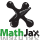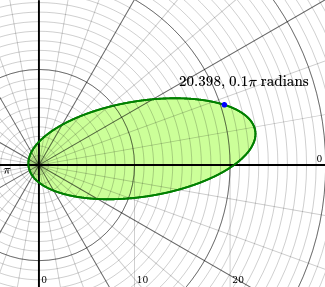SVG Graph

SVG Graph is a script library that creates mathematical graphs with Cartesian, logarithmic, or polar coordinates. Shapes and text labels may be added using the actual graph coordinates, centered on the origin. One may pan and zoom the graph, and use the resulting coordinates to fine-tune the graph itself. Graphs may be saved as inline or external .svg files, or .png images.

The interactive graphs below get an inset border when the mouse enters. Left-drag to pan, right-drag to zoom, double-click to reset. See more options and the output field at the bottom of the page.

Area under a parabola$$% MathJax is loading . . . . y = x^2 / 3$$

Exponential curve on semi-log coordinates

$$y = 16 ^ x$$

Polar coordinates

$$a = 12, ~ b = 5, ~ \phi = 9°, ~ e = \sqrt{ 1 - (b/a)^2}$$

$$\displaystyle r = \frac {a\,(1 - e^2)} { 1 - e \, \textrm{cos}(\theta - \phi) }$$

Bell curve

mean μ: 0.0

standard deviation σ: 1.0

area under 2.0 standard deviation(s):  %

Standard illuminants

|

Draw a rose

a: 2

b: 9   draw

$$r = \textrm{sin}(\frac{a}{b}\theta)$$

I assume this is hemp

$$r=(1+0.9 \cos(8 \theta)) (1+0.1 \cos(24 \theta)) (0.9+0.05 \cos(200 \theta)) (1+\sin(\theta))$$

 Interactive mouse and keyboard events drag: left pan left + H pan horizontally left + V pan vertically right zoom right + C zoom from center right + L zoom from lower left left + R resize canvas right + R resize contents both + R resize everything left click while holding: C center origin L origin to lower left H save HTML to the textarea P save parameters to the textarea T save tooltip to the textarea D redraw graph with parameters from the textarea (id’s must match) S save graph as png double click: reset press [ to enable the tooltip, ] to hide  (x or r, y or theta | xMin, xMax, yMin, yMax | ww × hh)

The files

svgGraph.zip, 16.3 KB

containing svgGraph.js, svgGraph.css, an svgGraph.htm sample page, and MathJax configuration and css files.

See svgGraph.js for documentation.

Examples

 inline svg: 10 20 0 0 π 20.398, 0.1π radians 14.762, 6.2686 | -4.1754, 29.825, -12.431, 17.569 | 325 × 286.76 #polar { background-color: white } #polar text { font-family: MathJax_Math } #polar .mjm { font-family: MathJax_Main } external svg:png:Please visit russellcottrell.com | blog